# Overview

The nph package includes functions to model survival distributions in terms of piecewise constant hazards and to simulate data from the specified distributions.

# Installation

The package is available from CRAN and can be installed directly from R.

install.packages("nph")

# For dev version
# install.packages("devtools")
devtools::install_github("repo/nph")

# Getting started

Basically, there are three mechanisms for non-proportionality available in this package:

• Disease progression
• Different effect by time intervals
• Subgroups

These scenarios are illustrated in the following figures. Note that the hazard ratio is not constant across time.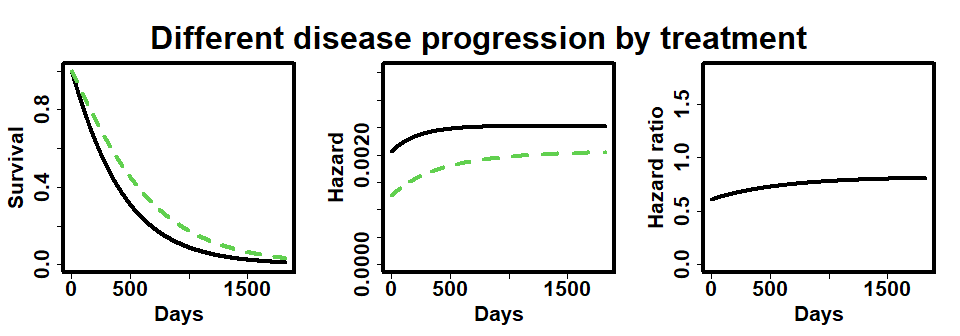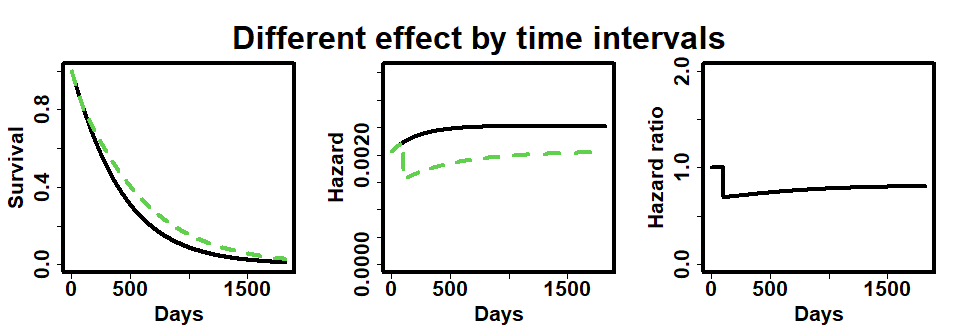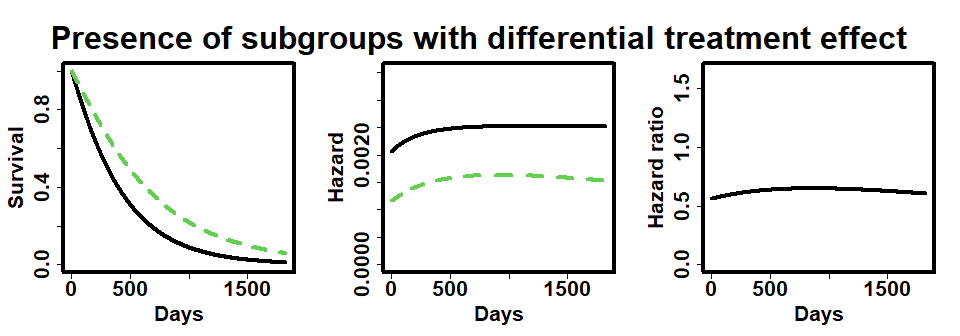# Basics

The functions of the package can be grouped according to their functionality.

Functions for modelling/setting the underlying survival model:

• hazVFfun
• subpop_pchaz
• pop_pchaz

Functions for generating simulated dataset given for a specified survival model:

• sample_fun
• sample_conditional_fun

Functions for performing statistical tests:

• logrank.test
• logrank.maxtest

Plotting functions:

• plot.mixpch
• plot_diagram
• plot_shhr

The basic underlying model for the survival mechanism assumes that each patient can be in one of three states: Alive with no progression of disease, Alive with progression of disease, and Dead.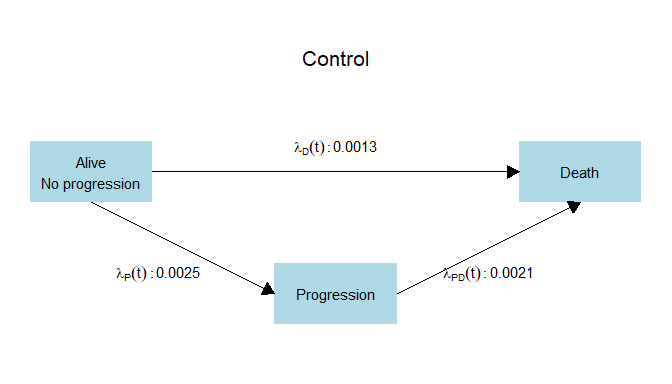## Creating the population model with pop_pchaz

The first step is to create the population model with the pop_pchaz function. As the previous figure shows, there are three hazard rates that need to be defined: the hazard of disease progression $$\lambda_P(t)$$, the hazard of death given no progression $$\lambda_P(t)$$, and the hazard of death given progression $$\lambda_P(t)$$. The arguments lambdaProgMat, lambdaMat1, and lambdaMat2 in the pop_pchaz function correspond to the three hazard rates, respectively.

The hazard rates are assumed piecewise constant functions across $$k$$ time intervals $$[t_{j-1}, t_j)$$, $$j=1, \ldots,k$$ with $$0=t_0<t_1<\ldots<t_k=\infty$$. Therefore, the pop_pchaz function has also an argument T that is a vector to specify $$t_0,t_1,\ldots,t_k$$. When T is of length 2 (and therefore only one time interval) lambdaProgMat, lambdaMat1, and lambdaMat2 are scalars. If T is of length > 2, then the lambdaProgMat, lambdaMat1, and lambdaMat2 are matrices where the number of columns is equal to the number of time intervals $\begin{bmatrix}\lambda^{[t_0-t_1)} & \lambda^{[t_1-t_2)} & \ldots &\lambda^{[t_{k-1}-t_k)}\end{bmatrix}.$

For example, if the patients are followed for two years but the hazards change after the first year, then T should be specified as c(0, 365, 2*365). If we assume a hazard rate for death of 0.02 and 0.04 for the first and second year respectively, the we should specify lambdaMat1 = matrix(c(0.02, 0.04), ncol = 2).

Finally, is is also possible to specify different hazard rates for subgroups. The pop_pchaz has the argument p which is intended to specify the subgroup prevalences. Given $$m$$ subgroups with relative sizes $$p_1, p_2, \ldots, p_m$$, then the p argument should be specified as c(p_1, p_2, ..., p_m). The lambdaProgMat, lambdaMat1, and lambdaMat2 then should have the number of row equal to the number of defined subgroups:

$\begin{bmatrix} \lambda_1 \\ \lambda_2 \\ \ldots \\ \lambda_m \end{bmatrix}.$

For example, if patients can be divided into two subgroup with prevalences 0.2 and 0.8 with hazard rates a hazard rate for death of 0.02 and 0.03 thoughout a one year interval, then we define T = c(0, 365), p = c(0.2, 0.8) and lambdaMat1 = matrix(c(0.02, 0.03), nrow = 2).

Naturally, it is possible to combine multiple time intervals and subgroups, then the hazard matrices have the form: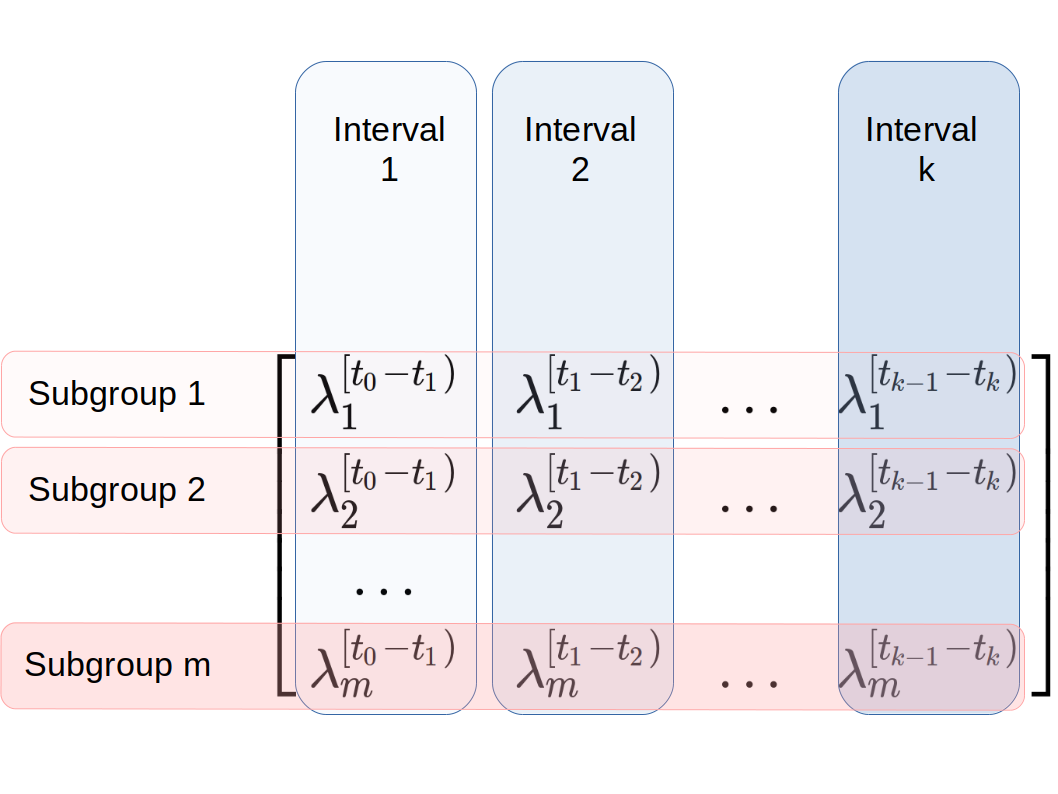Below, we consider an example where there two subgroups and two time intervals. In practice, this situation correspond to the case where there is a delayed effect of the drug. Note that for specifying the hazard matrices, we use the median time to death/progression and use the function m2r (also provided in the package) to obtain the hazard rates.

times <- c(0, 100, 5 * 365)   # Time interval boundaries, in days
t_resp <- c(0.2, 0.8) #Proportion of subgroups
B5 <- pop_pchaz(
T = times,
lambdaMat1    = m2r(matrix(c(11, 30,
11, 18), byrow = TRUE, nrow = 2)),
lambdaMat2    = m2r(matrix(c( 9, 20,
9, 11), byrow = TRUE, nrow = 2)),
lambdaProgMat = m2r(matrix(c( 5, 15,
5,  9), byrow = TRUE, nrow = 2)),
p = t_resp, discrete_approximation = TRUE
)

The results object is of class mixpch, which has a dedicated plotting function to visualize the survival and hazard functions.

plot(B5, main = "Survival function")
plot(B5, fun = "haz", main = "Hazard function")
plot(B5, fun = "cumhaz", main = "Cumulative Hazard function")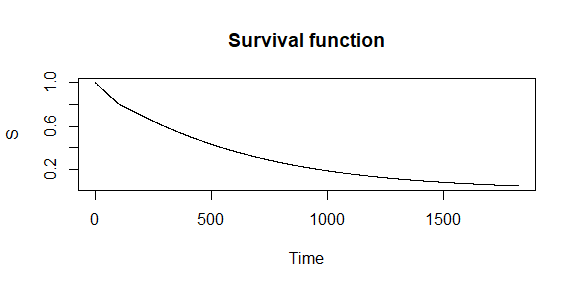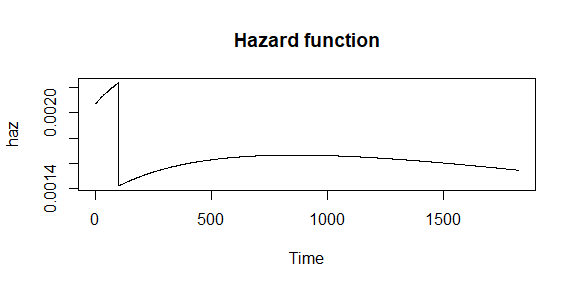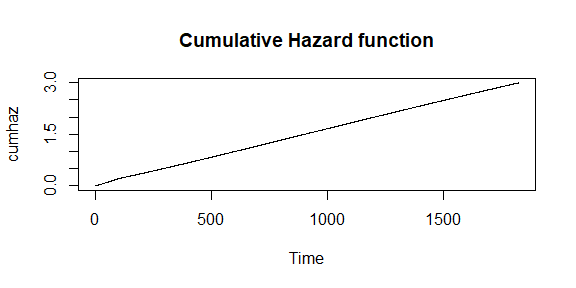## Creating a simulated dataset with sample_fun

The sample_fun function is designed to generate a simulated dataset that would be obtained from a parallel group randomised clinical trial.

The first step is to create two objects with the (theoretical) survival functions for the treatment and control groups using pop_pchaz:

times <- c(0, 100, 5 * 365)   # Time interval boundaries, in days
# Treatment group
B5 <- pop_pchaz(T = times,
lambdaMat1    = m2r(matrix(c(11, 30,
11, 18), byrow = TRUE, nrow = 2)),
lambdaMat2    = m2r(matrix(c( 9, 20,
9, 11), byrow = TRUE, nrow = 2)),
lambdaProgMat = m2r(matrix(c( 5, 15,
5,  9), byrow = TRUE, nrow = 2)),
p = c(0.2, 0.8),#Proportion of subgroups
discrete_approximation = TRUE
)
# Control group
K5  <- pop_pchaz(T = times,
lambdaMat1    = m2r(matrix(c(11, 11), nrow = 1)),
lambdaMat2    = m2r(matrix(c( 9,  9), nrow = 1)),
lambdaProgMat = m2r(matrix(c( 5,  5), nrow = 1)),
p = 1, discrete_approximation = TRUE
)

Then, using the resulting objects, we use them to generate a dataset with the sample_fun function:

# Study set up and Simulation of a data set until interim analysis at 150 events
set.seed(15657)
dat <- sample_fun(K5, B5,
r0 = 0.5,                     # Allocation ratio
eventEnd = 450,               # maximal number of events
lambdaRecr = 300 / 365,       # recruitment rate per day (Poisson assumption)
lambdaCens = 0.013 / 365,     # censoring rate per day  (Exponential assumption)
maxRecrCalendarTime = 3 * 365,# Maximal duration of recruitment
maxCalendar = 4 * 365.25)     # Maximal study duration
#>     group inclusion  y yCalendar event adminCens cumEvents
#> 777     1        26  3        29  TRUE     FALSE         1
#> 17      1        25 18        43  TRUE     FALSE         2
#> 367     1         9 40        49  TRUE     FALSE         3
#> 64      0        42  9        51  TRUE     FALSE         4
#> 25      0        34 40        74  TRUE     FALSE         5
#> 708     1       103  7       110  TRUE     FALSE         6
tail(dat)
#>     group inclusion   y yCalendar event adminCens cumEvents
#> 889     1       755 207       962 FALSE      TRUE       450
#> 893     0       205 757       962 FALSE      TRUE       450
#> 896     0       814 148       962 FALSE      TRUE       450
#> 900     1       510 452       962 FALSE      TRUE       450
#> 907     1       225 737       962 FALSE      TRUE       450
#> 908     0       717 245       962 FALSE      TRUE       450

## The weighted log-rank test and the max-LRtest

The weighted log-rank test is implemented using the function logrank.test, which uses the statistic:

$z = \sum_{t\in\mathcal{D}} w(t)(d_{t, ctr} - e_{t,ctr}) / \sqrt{\sum_{t\in\mathcal{D}} w(t)^2 var(d_{t, ctr})}.$

where $$w(t)$$ are the Fleming-Harrington $$\rho-\gamma$$ family weights, such that $$w(t)=\widehat{S}(t)^{\rho}(1-\widehat{S}(t))^{\gamma}$$. Under the the least favorable configuration in $$H_0$$, the test statistic is asymptotically standard normally distributed and large values of $$z$$ are in favor of the alternative.

For example, the following code performs the weighted log-rank test using the simulated dataset and $$\rho = 1$$ and $$\gamma = 0$$.

logrank.test(time  = dat$y, event = dat$event,
group = dat$group, # alternative = "greater", rho = 1, gamma = 0) #> Call: #> logrank.test(time = dat$y, event = dat$event, group = dat$group,
#>     rho = 1, gamma = 0)
#>
#>     N Observed Expected (O-E)^2/E (O-E)^2/V
#> 1 411      267      211      15.2      57.8
#> 2 385      183      239      13.3      57.8
#>
#>  Chisq= 22.1  on 1 degrees of freedom, p= 3e-06
#>  rho   =  1 gamma =  0
# survival::survdiff(formula = survival::Surv(time  = dat$y, event = dat$event) ~ dat$group) For a set of $$k$$ different weight functions $$w_1(t), \ldots, w_k(t)$$, the maximum log-rank test statistic is $$z_{max} = \max_{i=1,\ldots,k}z_i$$. Under the least favorable configuration in $$H_0$$, approximately $$(Z_1, \ldots, Z_k) \sim N_k(0, \Sigma)$$. The $$p$$-value of the maximum test, $$P_{H_0}(Z_{max} > z_{max})$$, is calculated based on this multivariate normal approximation via numeric integration. The following code performs the maximum log-rank test using four combinations of $$\rho$$ and $$\gamma$$ for the weights. lrmt = logrank.maxtest( time = dat$y,
event = dat$event, group = dat$group,
rho   = c(0, 0, 1, 1),
gamma = c(0, 1, 0, 1)
)
lrmt
#> Call:
#> logrank.maxtest(time = dat$y, event = dat$event, group = dat$group, #> rho = c(0, 0, 1, 1), gamma = c(0, 1, 0, 1)) #> #> Two sided p-value = 5.09e-08 (Bonferroni corrected: 2.03e-07) #> #> Individual weighted log-rank tests: #> Test z p #> 1 1 5.37 7.99e-08 #> 2 2 5.23 1.73e-07 #> 3 3 4.70 2.63e-06 #> 4 4 5.45 5.08e-08 The individual tests can also be accesed using the testListe elements in the resulting object. lrmt$logrank.test[]
#> Call:
#> logrank.test(time = time, event = event, group = group, alternative = alternative,
#>     rho = rho[i], gamma = gamma[i])
#>
#>     N Observed Expected (O-E)^2/E (O-E)^2/V
#> 1 411      267      211      15.2      28.8
#> 2 385      183      239      13.3      28.8
#>
#>  Chisq= 28.8  on 1 degrees of freedom, p= 8e-08
#>  rho   =  0 gamma =  0
lrmt$logrank.test[] #> Call: #> logrank.test(time = time, event = event, group = group, alternative = alternative, #> rho = rho[i], gamma = gamma[i]) #> #> N Observed Expected (O-E)^2/E (O-E)^2/V #> 1 411 267 211 15.2 187 #> 2 385 183 239 13.3 187 #> #> Chisq= 27.3 on 1 degrees of freedom, p= 2e-07 #> rho = 0 gamma = 1 lrmt$logrank.test[]
#> Call:
#> logrank.test(time = time, event = event, group = group, alternative = alternative,
#>     rho = rho[i], gamma = gamma[i])
#>
#>     N Observed Expected (O-E)^2/E (O-E)^2/V
#> 1 411      267      211      15.2      57.8
#> 2 385      183      239      13.3      57.8
#>
#>  Chisq= 22.1  on 1 degrees of freedom, p= 3e-06
#>  rho   =  1 gamma =  0
lrmt\$logrank.test[]
#> Call:
#> logrank.test(time = time, event = event, group = group, alternative = alternative,
#>     rho = rho[i], gamma = gamma[i])
#>
#>     N Observed Expected (O-E)^2/E (O-E)^2/V
#> 1 411      267      211      15.2       810
#> 2 385      183      239      13.3       810
#>
#>  Chisq= 29.7  on 1 degrees of freedom, p= 5e-08
#>  rho   =  1 gamma =  1